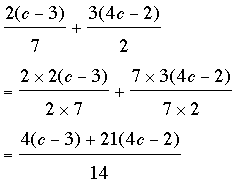SEARCH HOMEMath Central Quandaries & QueriesQuestion from amanpreet, a student: please can you help me to simplify: 2(c-3) / 7 + 3(4c-2)/2Amanpreet,

To add two fractions you need a common denominator. The denominators of these fractions are 2 and 7 so a common denominator is 2 × 7 = 14. ThusSimplify the numerator.

PennyMath Central is supported by the University of Regina and The Pacific Institute for the Mathematical Sciences.